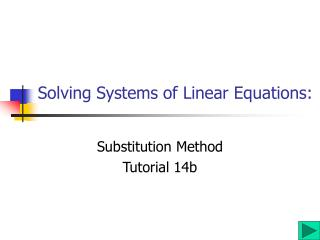DownloadDownload PresentationSolving Systems of Linear Equations:

# Solving Systems of Linear Equations:

Download Presentation## Solving Systems of Linear Equations:

- - - - - - - - - - - - - - - - - - - - - - - - - - - E N D - - - - - - - - - - - - - - - - - - - - - - - - - - -
##### Presentation Transcript

1. Solving Systems of Linear Equations: Substitution Method Tutorial 14b

2. 3 Methods to Solve • There are 3 methods that we can use to solve systems of linear equations. • Solve by the Graphing Method • Solve by the Substitution Method • Solve by the Addition (Elimination) Method

3. -10 -10 Substitution Method Solve x + 3y = 7 and 2x – 4y = -6. x + 3y = 7 • Solve one equation for one variable. • The first equation would be easiest since the x term already has a coefficient of one. -3y -3y x = 7 – 3y 2x – 4y = -6 • Substitute 7 –3y for x in the second equation. Then solve for y. 2(7 – 3y) – 4y = -6 • Substitute 2 for y in either one of the two original equations to find the value of the x coordinate. 14 – 6y – 4y = -6 14 – 10y = -6 -14 -14 x +3(2) = 7 x + 6 = 7 x = 1 • Step 4 is to check the answer. Click here! 2 1 -10y = -20 y = 2 1 1

4. Substitution Method Solve x + 3y = 7 and 2x – 4y = -6. • The last step is to check your solution by substituting both values, x = 1 & y = 2, in both equations. x + 3y = 7 1 +3(2) = 7 1 + 6 = 7 7 = 7  2x – 4y = -6 2(1) – 4(2) = -6 2 – 8 = -6 -6 = -6  The solution of the system is (1, 2).

5. Substitution Method: In Review Steps for the Substitution Method • Solve one equation for one of the variables. • Substitute the resulting expression in the other equation. Solve that equation. • Substitute the value of the variable from step 2 in either equation. Solve the resulting expression. • Check by substituting both values in both equations.

6. The End Time to move on to the assignment or the next lesson